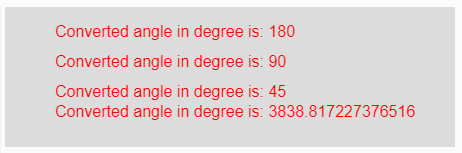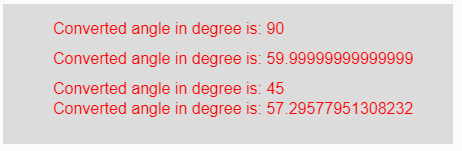Open in App
Not now

# p5.js | degrees() function

• Last Updated : 22 Apr, 2019

The degrees() function in p5.js is used to convert a given radian measurement value to its corresponding value in degrees.

Syntax:

`degrees( radian )`

Parameters: This function accepts single parameter radian which is to be converted into degrees.

Return Value: It returns the converted angle into degrees.

Below programs illustrate the degrees() function in p5.js:

Example 1: This example uses degrees() function to convert given radian measurement value to its corresponding value in degrees.

 `function` `setup() {  ` `  `  `    ``// Creating Canvas size ` `    ``createCanvas(450, 140);  ` `}  ` ` `  `function` `draw() {  ` `      `  `    ``// Set the background color  ` `    ``background(220);  ` `    `  `    ``// Initializing some angles in radians ` `    ``let Rad1 = PI;  ` `    ``let Rad2 = PI / 2;  ` `    ``let Rad3 = PI / 4;  ` `    ``let Rad4 = 67;  ` `     `  `     `  `    ``// Calling to degrees() function ` `    ``let A = degrees(Rad1); ` `    ``let B = degrees(Rad2); ` `    ``let C = degrees(Rad3); ` `    ``let D = degrees(Rad4); ` `     `  `    ``// Set the size of text  ` `    ``textSize(16);  ` `      `  `    ``// Set the text color  ` `    ``fill(color(``'red'``));  ` `    `  `    ``// Getting converted angles into degree ` `    ``text(``"Converted angle in degree is: "` `+ A, 50, 30); ` `    ``text(``"Converted angle in degree is: "` `+ B, 50, 60); ` `    ``text(``"Converted angle in degree is: "` `+ C, 50, 90); ` `    ``text(``"Converted angle in degree is: "` `+ D, 50, 110); ` `}  `

Output:Example 2: This example uses degrees() function to convert given radian measurement value to its corresponding value in degrees.

 `function` `setup() {  ` `  `  `    ``// Creating Canvas size ` `    ``createCanvas(450, 140);  ` `}  ` ` `  `function` `draw() {  ` `      `  `    ``// Set the background color  ` `    ``background(220);  ` `     `  `    ``// Calling to degrees() function with different ` `    ``// radians value as parameter ` `    ``let A = degrees(PI/2); ` `    ``let B = degrees(PI/3); ` `    ``let C = degrees(PI/4); ` `    ``let D = degrees(PI/PI); ` `     `  `    ``// Set the size of text  ` `    ``textSize(16);  ` `      `  `    ``// Set the text color  ` `    ``fill(color(``'red'``));  ` `    `  `    ``// Getting converted angles into degree ` `    ``text(``"Converted angle in degree is: "` `+ A, 50, 30); ` `    ``text(``"Converted angle in degree is: "` `+ B, 50, 60); ` `    ``text(``"Converted angle in degree is: "` `+ C, 50, 90); ` `    ``text(``"Converted angle in degree is: "` `+ D, 50, 110); ` `}   `

Output:Reference: https://p5js.org/reference/#/p5/degrees

My Personal Notes arrow_drop_up
Related Articles